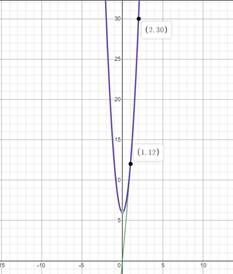# Solution of given inequality by appropriate graph.### Precalculus: Mathematics for Calcu...

6th Edition
Stewart + 5 others
Publisher: Cengage Learning
ISBN: 9780840068071### Precalculus: Mathematics for Calcu...

6th Edition
Stewart + 5 others
Publisher: Cengage Learning
ISBN: 9780840068071

#### Solutions

Chapter 1.9, Problem 61E
To determine

## Solution of given inequality by appropriate graph.

Expert Solution

x[1,2]

### Explanation of Solution

Given information:

x3+11x6x2+6

Formula used:

Solving by making graph.

Graphically:

Making Graph of y=x3+11x and y=6x2+6 on one viewing rectangle.As per the given inequality, the region where y=x3+11x (Green line) lies below the line of y=6x2+6 (Purple Line) and their intersection points are required solutions:

x[1,2]

### Have a homework question?

Subscribe to bartleby learn! Ask subject matter experts 30 homework questions each month. Plus, you’ll have access to millions of step-by-step textbook answers!